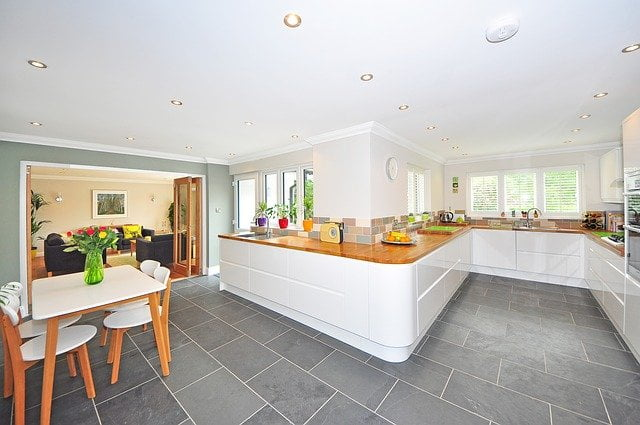## how to estimate the cost of tiling a room

how to estimate the cost of tiling a room

Today we’ll show you the process of estimating the cost of tiling a room and we are going to do it step by step so that by the end of the article you’re able to determine the number of tiles, Cement, sand required for the tile flooring work and the cost of associated labor component. I will explain you in detail. Let us consider a room 5m and length of 12m. The standard size of one tile is considered as 0.4m x 0.4m. Let us consider the thickness of flooring as 50mm.The size of one tile is considered as 0.4 meters by 0.4 meters.

= 0.4 x 0.4

= 0.16 sq meters

For the calculation of the area of the room to be floored multiply the width of room and length of the room. That is this 5m x 12m so the workout is presented below.

Floor area = floor width x floor length

= 5 x 12

= 60 sq meters

The proportion of mortar is 1:4 where1 is cement and 4 is sand.

Contents

# STEP 1

### Calculating Wet volume

First, let’s calculate the wet volume of mortar that will be required for the bed of flooring. So the formula will thickness of flooring into the area of flooring.

Wet volume = floor area x thickness of the flooring

But remember that we have taken the thickness of flooring in millimeters so to convert it into a meter we will have to divide that value by 1000 first.

Given the thickness of our flooring as 50 mm.

= 50/1000 meters

= 0.05 meters

Wet volume = 60 x 0.05 cubic meters

= 3 cubic meters

# STEP 2

### Calculating Dry volume

The dry volume of mortar is considered as 25% more than the wet volume of mortar therefore to calculate the dry volume of mortar;

Dry volume = Wet volume x (100+25)%

= 3 x 125%

= 3 x 1.25

= 3.75 cubic meters

The next step is to calculate the quantity of cement required

# STEP 3

### Calculating Quantity of Cement Required.

The formula is dry volume divided by the sum of ratio times proportion of cement that is this

Cement Quantity = (Dry volume/(sum of ratio))x(Proportion of Cement)

Given the ratio cement:sand as 1:4

sum of ratio = 1+4

= 5

Cement Quantity =( 3.75/(5)x1)

= 3.75/5

= 0.75 cubic meters

# STEP 4

### Calculating Bags of Cement Required

To calculate the number of bags of cement required we know that the volume of 1 bag of 50 kilograms cement bag is 0.035 cubic meter therefore use formulas is

Bags of Cement = (Quantity of Cement) / (Volume of 1 bag)

= 0.75/0.035

= 21.43

= 22

(22 bags after Roundup)

Roundup is important because the cement bags cannot be taken into points therefore roundup that value to the next round figure

# STEP 5

### Calculating quantity of Sand required

Sand Quantity = (Dry volume/(sum of ratio))x(Proportion of Sand)

= (3.75/(5))x4

= 15/5

= 3 cubic meters

# STEP 6

### Calculating the Number of Tiles required

Number of Tiles = Area of Flooring/Size of one tile

= (5×12)/(0.4×0.4)

= 375

# STEP 7

### calculate the total cost of materials

We’ll use the Goodwill Floor Tile, flooring, and polishing.

We have taken the rate of one bag of cement as 30,000/= and Consiecidentally, the sand loading capacity of a 5ton Isuzu Forward Truck is about 3 cubic meters which is the exact quantity required as per our example and the cost per trip is about Ugx. 210,000/=. Therefore, we’ll take the rate of sand as 70,000/= per cubic meter.

The current rate for Goodwill Floor Tile in Uganda is about 22,800/= per square meter, and we know there are (6.25 pieces) Per Square Meter. If we divide the cost per square meter(22,800) by the number of pieces in a square meter(6.25), we can safely round up the cost per piece to 3648/=.

The rate of laying and polishing is considered as 10,000/= per square meter.

### total cost of Goodwill Floor Tile (C1)

= (Total Number of tiles) x (cost per piece)

= 375 x 3648

C1 = 1,368,000/=

NB: This could also be calculated using total floor area(60 sq meters) by cost of tiles per square meter(22,800) i.e. 60 x 22800 = 1,368,000/=

### total cost of laying & polishing (C2)

= (Total floor area) x (Labor rate per square meter)

= 60 x 10000

C2 = 600,000/=

### total cost of cement bags (C3) =

(Number of total bag) x (cost per bag)

= 22 x 30,000

C3 = 660,000/=

### total cost of sand (C4) =

(quantity of sand) x (cost per cubic meter)

= 3 x 70,000

C4 = 210,000/=

Now we can calculate the total cost involved; which is the summation of the above

### Total cost

= C1+C2+C3+C4

= 1,368,000 + 600,000 + 660,000 + 210,000

= 2,838,000/=